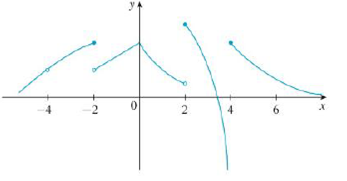Chapter 2.5, Problem 3E

Chapter
Section
Textbook Problem

(a) From the graph of f , state the numbers at which f is discontinuous and explain why.(b) For each of the numbers stated in part (a), determine whether f is continuous from the right, or from the left. or neither.(a)

To determine

To state: The numbers at which f is discontinuous from the graph.

Explanation

Definition used: “A function f is continuous at a number a if limxaf(x)=f(a)”.

Note 1: “If f is defined near a, f is discontinuous at a whenever f is not continuous at a

(b)

To determine

Whether f is continuous from the right, or from the left, or neither at the numbers −4, −2, 2 and 4.

Still sussing out bartleby?

Check out a sample textbook solution.

See a sample solution

The Solution to Your Study Problems

Bartleby provides explanations to thousands of textbook problems written by our experts, many with advanced degrees!

Get Started

Find f'(a). f(x) = 3x2 4x + 1

Single Variable Calculus: Early Transcendentals, Volume I

In Exercises 43-46, find f(a + h) f(a) for each function. Simplify yoiir answer. 44. f(x)=12x+3

Applied Calculus for the Managerial, Life, and Social Sciences: A Brief Approach

Find the slope of each line:

Elementary Technical Mathematics

What are the two requirements for a random sample?

Statistics for The Behavioral Sciences (MindTap Course List)

Given: Line l and point P not on l Construct: PQl

Elementary Geometry for College Students

In the graph at the right; limx2f(x)=. a) 2 b) 4 c) 0 d) does not exist

Study Guide for Stewart's Single Variable Calculus: Early Transcendentals, 8th